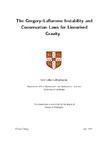## The Gregory--Laflamme Instability and Conservation Laws for Linearised Gravity

##### Authors
Collingbourne, Sam
##### DOIThesis
##### Abstract

This thesis is concerned with the black hole stability problem in general relativity. In particular, it presents stability and instability results associated to the linearised vacuum Einstein equation on black hole backgrounds.

The first chapter of this thesis gives a direct rigorous mathematical proof of the Gregory--Laflamme instability for the 5-dimensional Schwarzschild black string. Under a choice of ansatz for the perturbation and a gauge choice, the linearised vacuum Einstein equation reduces to an ODE problem for a single function. In this work, the ODE is cast into a Schrödinger eigenvalue equation to which an energy functional is assigned. It is then shown by direct variational methods that the lowest eigenfunction gives rise to an exponentially growing mode solution which has admissible behaviour at the future event horizon and spacelike infinity. After the addition of a pure gauge solution, this gives rise to a regular exponentially growing mode solution of the linearised vacuum Einstein equation in harmonic/transverse-traceless gauge.

The remainder of this thesis is concerned with conservation laws associated to the linearised vacuum Einstein equation. For later application, chapter 2 of this thesis contains a review of the double null gauge for the vacuum Einstein equations. In chapter 3, the `canonical energy' conservation law of Hollands and Wald is studied. This canonical energy conservation law gives an appealing criterion for stability of black holes based upon a conserved current. The method is appealing in its simplicity as it requires one to 'simply’ check the sign of the canonical energy Ε with Ε>0 implying weak stability and Ε<0 implying instability. However, in practice establishing the sign of E proves difficult. Indeed, even for the 4-dimensional Schwarzschild black hole exterior the positivity was not previously established. In this thesis, a resolution to this issue for the Schwarzschild black hole is presented by connecting to another weak stability result of Holzegel which exploits the double null gauge. Further weak stability statements for the Schwarzschild black hole (including a proof of mode stability) arising from the canonical energy are also established.

In chapter 4, some preliminary results associated to a novel conserved current associated to the linearised vacuum Einstein equation are presented. This can be viewed as a modification/simplification of the conserved current associated to the canonical energy. In particular, applications of this current to other black hole spacetimes are discussed.

2022-04-28